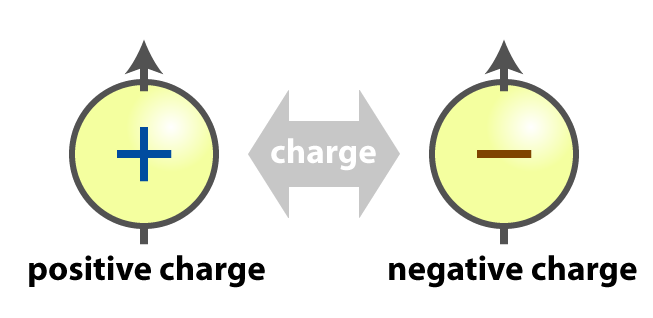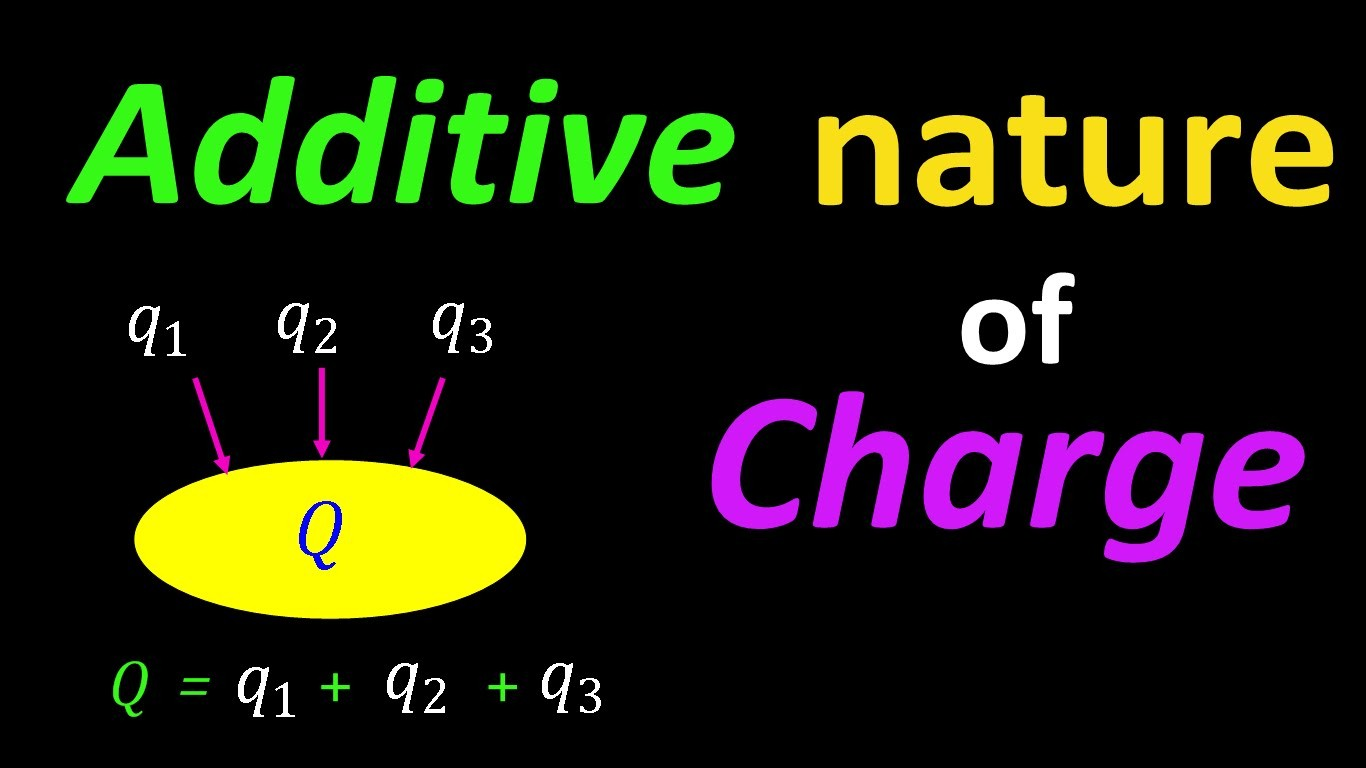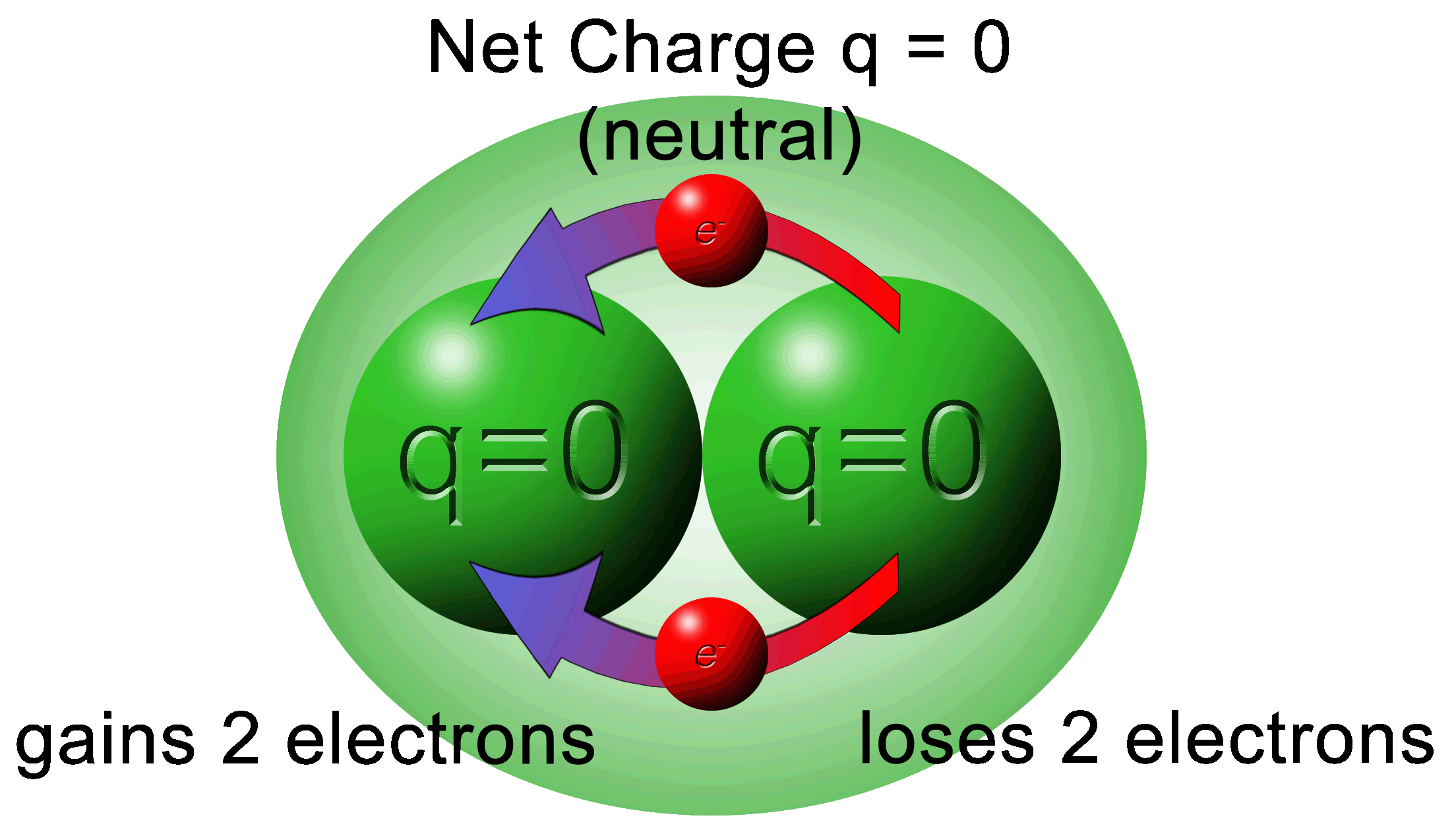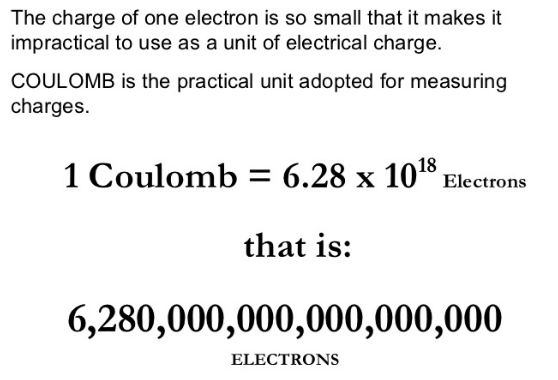Click to Chat

1800-1023-196

+91-120-4616500

CART 0

• 0

MY CART (5)

Use Coupon: CART20 and get 20% off on all online Study Material

ITEM
DETAILS
MRP
DISCOUNT
FINAL PRICE
Total Price: Rs.

There are no items in this cart.
Continue Shopping• Complete Physics Course - Class 11
• OFFERED PRICE: Rs. 2,968
• View Details

```Basic Properties of Electric Charge

Table of Content

Electric Charge

Unit of Charge

Basic Properties of Electric Charge

Charge is a conserved quantity

Quantization of Charge

Electric ChargeImage 1: Types of charges

Electric Charge is defined as the amount of energy or electrons passing from one body to another either by conduction, induction or other specific methods. There are two types of electric charges namely positive charge and negative charges. Charges are present in almost everybody and those bodies which don’t have charges are assumed to be neutrally charged or no charge.

The charge is denoted by the symbol ‘q’ and its standard unit is Coulomb. Mathematically charge is the number of electrons multiplied by the charge on 1 electron. That is

Q = ne

where q is a charge, n is a number of electrons and e is a charge on 1 electron (1.6 × 10-19C )Image 2: Basic nature of charges

Electric charge is a fundamental property of a particle just like its mass and length. It also signifies the force of attraction between two electrons.The two very basic natures of electric charges is

Like charges repel each other, that is one electron will repel another electron, or one proton will repel another proton

Unlike charges attract each other, that one electron will attract another proton or vice versa

The nature of charges is responsible for the forces acting on them and coordinating direction of flow of them. The charge on electron and proton is same in magnitude which is 1.6 × 10-19 C. The only difference is that we show charge on proton with a positive sign and on electron with a negative sign.

Unit of Charge

The S.I or Standard unit of electric charge is Coulomb. Its symbol is C and 1 C is defined as the charge flowing through a wire in 1 sec if the current flowing in the wire is 1 A. Charge of a body is measured by comparing it to a standard value.

Basic Properties of Electric Charge

As charges are of two types, positive and negative, there are other certain basic properties they follow. If the size of charged bodies is so small, we consider them as point charges. The basic properties of electric charges are as follows:

Charge is a conserved quantity

Quantization of chargeImage 3: Adding charges in a system

Charges are additive in nature means they’re like scalars and can be added directly. For An Example consider a system which consists of two charges namely q1 and q2. Now we wish to find the total charge of the system. The total charge of the system will be the algebraic sum of q1 and q2 i.e.q1 + q2. The same thing holds for a number of charges in a system. Let's say a system contains q1,q2,q3,q4……….qn, then the net charge of the entire system will be

= q1 + q2 + q3 + q4 + ………………………..+ qn

The charge is a scalar quantity as it has only magnitude and no direction. The charge is just as other fundamental properties of the system like mass. The only difference between mass and charge is that charge is both positive and negative, while mass is always positive.

Example:

The charges of a system are +3 C, + 2 C, + 5 C and -4 C respectively. What would be the net charge of the system?

We know that net charge of a system is algebraic sum of individual charges. Let the total charge of the system be “Q”. Then

Q = 3 C + 2 C + 5C – 4C

= 6 C

Charge is a conserved quantityImage 4: Charge is conserved

The charge is a conserved quantity which means charge can neither be created nor be destroyed but can be transferred from one body to another by certain methods like conduction and induction. As charging involves rubbing two bodies, it is actually a transfer of electrons from one body to another. We can’t create a charge in a body but eventually can transfer them to another body with some convenient methods.

In a system when charges are distributed accordingly, by the principle of conservation the net charge of the system remains constant. As an example if 5 C is the total charge of the system, then it can be redistributed as 1C, 2C and 2C or in any other possible permutation, but by conservation principle the net charge of system will always be 5 C. Although the charge carriers may be destroyed in a system but the net charge will remain conserved.

Example

Sometimes a neutrino decays to give one electron and one proton by default in nature. The net charge of the system will be zero as electrons and protons are of same magnitude and opposite signs. Then the net charge of the system before the creation of electron and proton (that is zero) equals to a net charge of the system after the creation of electron and proton (which is again zero). This proves the conservation principle.

Quantization of ChargeImage 5: Number of electrons in 1 Coulomb

Quantization of charge means that charge is a quantized quantity and is expressed as integral multiples of the basic unit of charge (e – charge on one electron). Suppose charge on a body is q, then it can be written as

q = ne

where n is an integer and not fraction or irrational number, like ‘n’ can be any positive or negative integer like 1, 2, 3, -5 etc.

The basic unit of charge is the charge acquired by an electron or proton. By convention we take charge on the electron as negative and denote it as “-e” and charge on a proton is simply “e”. The quantization of charge principle was first proposed by English experimentalist Faraday when he put forward his experimental laws of electrolysis. The principle was finally demonstrated and proved by Millikan in 1912.

1 A Coulomb of charge contains around 6 × 1018 electrons. Particles don’t have a high magnitude of charge and we use micro coulombs or milli coulombs in order to express charge of a particle.

1 μC = 10-6 C

1 mC = 10-3 C

The principle of quantization can be used to calculate the total amount of charge present in a body and also to calculate a number of electrons or protons in a body.

Suppose a system has n1 number of electrons and n2 number of protons, then total amount of charge will be n2e – n1e.

Watch this Video for more reference

Basic Properties of Electric Charge
```### Course Features

• 101 Video Lectures
• Revision Notes
• Previous Year Papers
• Mind Map
• Study Planner
• NCERT Solutions
• Discussion Forum
• Test paper with Video Solution